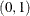# The QUANTLIFE Procedure

### MODEL Statement

Subsections:

• MODEL response <*censor(list)> = <effects> </ options>;

The MODEL statement identifies the response variable, the optional censoring variable, and the explanatory effects, including covariates, main effects, interactions, and nested effects; for more information, see the section Specification of Effects in Chapter 46: The GLM Procedure. In the MODEL statement, the response variable precedes the equal sign. This name can optionally be followed by an asterisk, the name of the censoring variable, and a list of censoring values (separated by blanks or commas if you list more than one value) enclosed in parentheses. If the censoring variable takes on one of these values, the corresponding failure time is considered to be censored. Following the equal sign are the explanatory effects (sometimes called independent variables or covariates) for the model.

The censoring variable must be numeric.

#### Options

You can specify the following options after a slash (/):

NOINT

specifies no intercept regression.

QUANTILE=number-list | PROCESS

specifies the quantile levels of interest for quantile regression analysis. You can specify any number of quantile levels in the interval. You can also compute the entire quantile process by specifying the PROCESS option.

If you do not specify the QUANTILE= option, the QUANTLIFE procedure fits a median regression, which corresponds to QUANTILE=0.5.